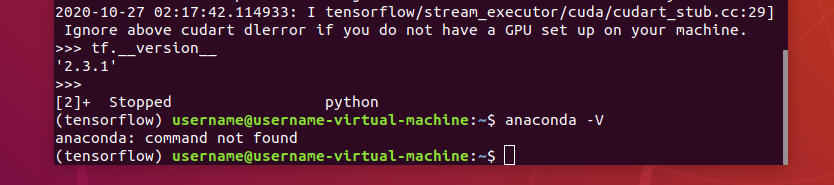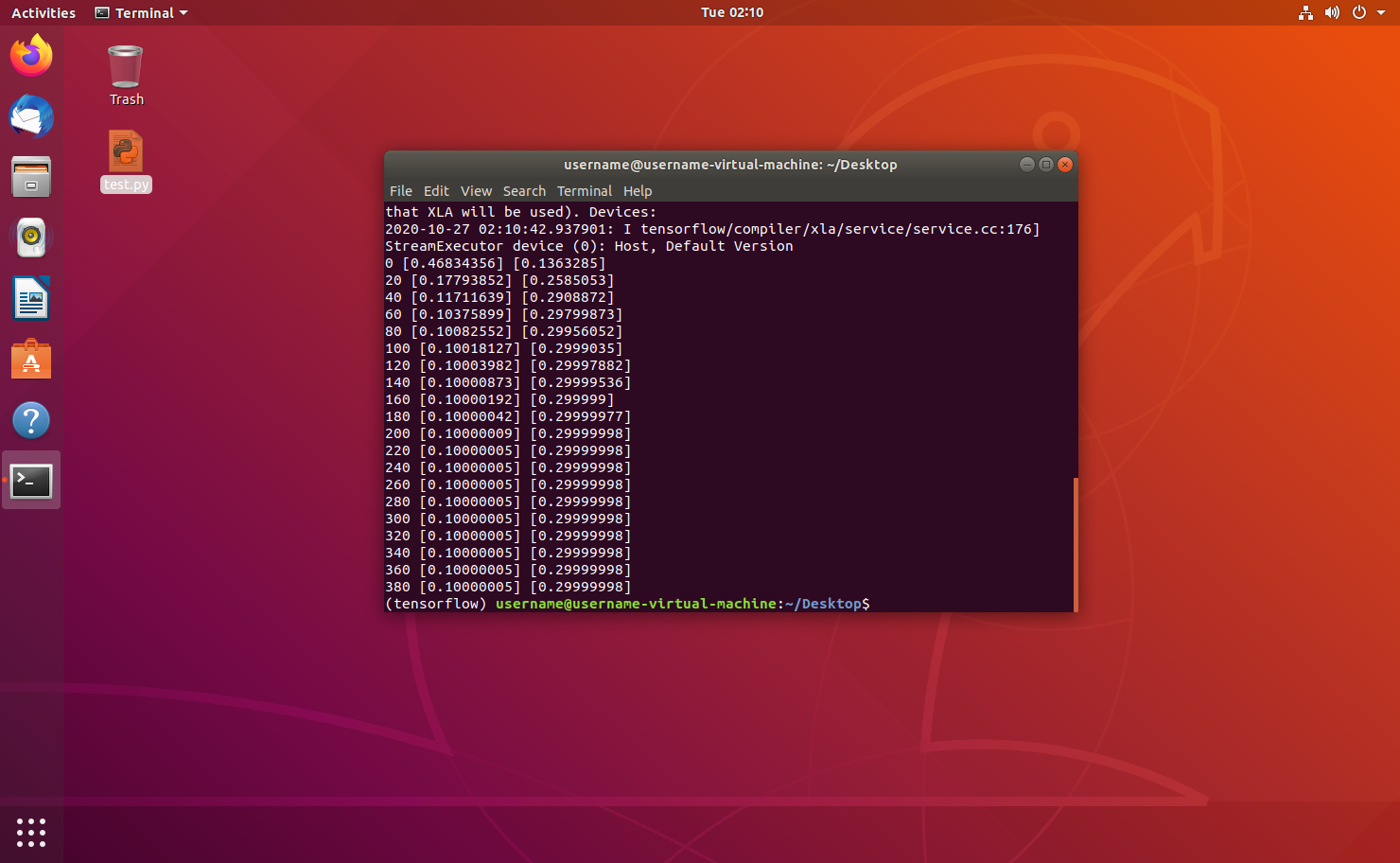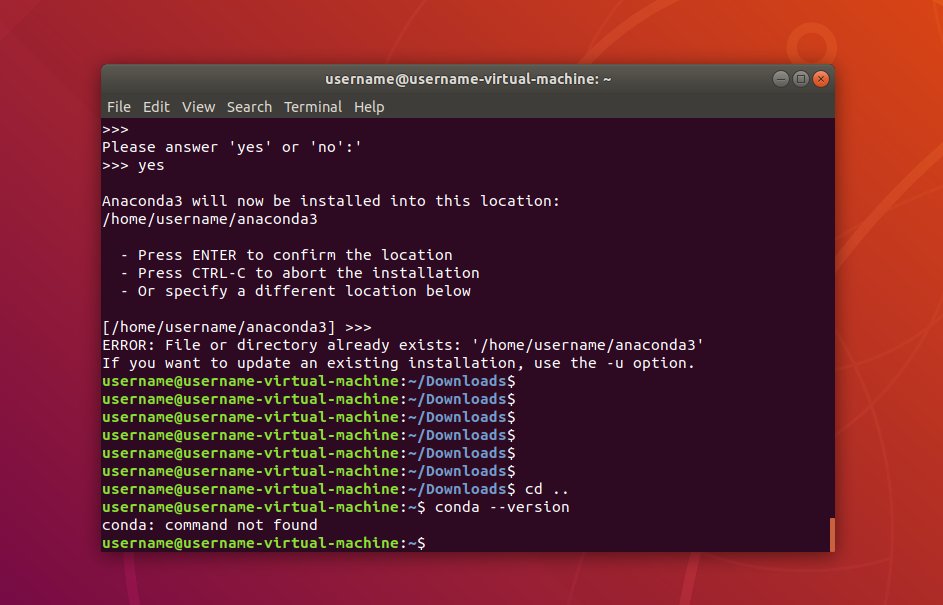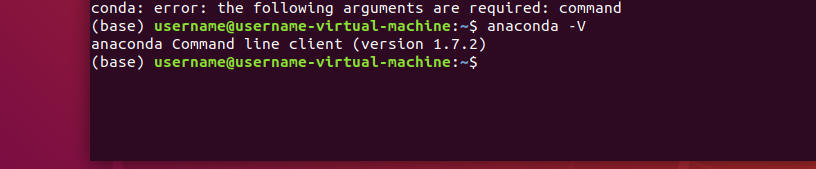# 1、 项目背景、功能需求分析

• 什么是逆概率
• 我们肯定知道正概率，举个例子就是，箱子里有5个黑球5个白球，那你随机拿到黑球和白球的概率都是50%，那现在我不知道箱子里有多少个黑球白球，那我通过不断的拿球应该如何确定箱子里有多少个黑球白球呢，这就是出名的逆概率
• 其实机器学习很多时候也就是逆概率的问题，我有大量现实例子的情况下，让机器从这些例子中找到共同的特征，例如给一万张猫的图片给机器学习，然后找到共同的特征（两只耳朵，四只脚，有胡须，有毛，有尾巴等特征）
• 根据逆概率的概念我们再举个其他场景
• y=Ax+B（A、B是常量）
• 如何通过这些x和y值来得到A和B的值？

# 2、 选题使用相关技术及环境

1.系统是ubuntu 18.04 LTS
2.我用的是pycharm来写的代码
3.Python用的是3.6.4
4.anaconda的版本为1.7.2
5.TensorFlow用的是2.3.1


# 3、 概要设计

pip install tensorflow -i https://pypi.douban.com/simple

import tensorflow as tf

tf.version# 4、详细设计与实现


##构造数据##
x_data=np.random.rand(100).astype(np.float32) #随机生成100个类型为float32的值
y_data=x_data*0.1+0.3  #定义方程式y=x_data*A+B
##-------##

##建立TensorFlow神经计算结构##
weight=tf.Variable(tf.random_uniform(,-1.0,1.0))
biases=tf.Variable(tf.zeros())
y=weight*x_data+biases
##-------##

loss=tf.reduce_mean(tf.square(y-y_data))  #判断与正确值的差距
train=optimizer.minimize(loss) #建立训练器

init=tf.initialize_all_variables() #初始化TensorFlow训练结构
sess=tf.Session()  #建立TensorFlow训练会话
sess.run(init)     #将训练结构装载到会话中



# 5、 系统测试# 6、总结

1、一开始下载好Ubuntu18.0.4很多东西都没有下载好，借助百度的力量一步步下载pip,vim，python

2、再者在安装anaconda的时候一直安装不成功anaconda历史版本

3、安装TensorFlow一直爆红，后面发现原来是网速不行，因为TensorFlow下载是用外网的，考虑到TensorFlow的文件太大，直接pip install tensorflow容易失败，所以直接使用清华园的镜像

pip install tensorflow -i https://pypi.douban.com/simple

4、在全部环境配置好的运行py文件一直出现这个错误

AttributeError: module ‘tensorflow’ has no attribute 'random_normal

import tensorflow.compat.v1 as tf

tf.disable_v2_behavior()

anaconda历史版本

ubuntu18.04 anaconda安装tensorflow

#导入依赖库
import numpy as np #这是Python的一种开源的数值计算扩展，非常强大
import tensorflow as tf  #导入tensorflow

##构造数据##
x_data=np.random.rand(100).astype(np.float32) #随机生成100个类型为float32的值
y_data=x_data*0.1+0.3  #定义方程式y=x_data*A+B
##-------##

##建立TensorFlow神经计算结构##
weight=tf.Variable(tf.random_uniform(,-1.0,1.0))
biases=tf.Variable(tf.zeros())
y=weight*x_data+biases
##-------##

loss=tf.reduce_mean(tf.square(y-y_data))  #判断与正确值的差距
train=optimizer.minimize(loss) #建立训练器

init=tf.initialize_all_variables() #初始化TensorFlow训练结构
sess=tf.Session()  #建立TensorFlow训练会话
sess.run(init)     #将训练结构装载到会话中

for  step in range(400): #循环训练400次
sess.run(train)  #使用训练器根据训练结构进行训练
if  step%20==0:  #每20次打印一次训练结果
print(step,sess.run(weight),sess.run(biases)) #训练次数，A值，B值


00次
sess.run(train) #使用训练器根据训练结构进行训练
if step%20==0: #每20次打印一次训练结果
print(step,sess.run(weight),sess.run(biases)) #训练次数，A值，B值



05-16
08-02120704-22
07-1146
06-01
04-2867
12-132万+
08-2867
04-234088
10-06